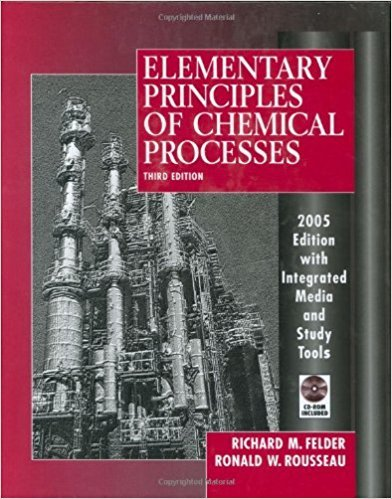×
Get Full Access to Elementary Principles Of Chemical Processes - 3 Edition - Chapter 8 - Problem 8.93
Get Full Access to Elementary Principles Of Chemical Processes - 3 Edition - Chapter 8 - Problem 8.93

×

# One g-mole of pure liquid sulfuric acid at temperature ToISBN: 9780471687573 143

## Solution for problem 8.93 Chapter 8

Elementary Principles of Chemical Processes | 3rd Edition

• Textbook Solutions
• 2901 Step-by-step solutions solved by professors and subject experts
• Get 24/7 help from StudySoup virtual teaching assistantsElementary Principles of Chemical Processes | 3rd Edition

4 5 1 297 Reviews
23
5
Problem 8.93

One g-mole of pure liquid sulfuric acid at temperature To (0C) is mixed with r g-moles of liquid water, also at temperature To(C), in an adiabatic container. The final solution temperature is Ts(C). The mass heat capacities of the pure acid, pure water, and the product solution [J/(g'0C)] are Cpa, Cpw , and Cps, respectively, all of which may be taken to be constant (independent of temperature). (a) Without doing any calculations, sketch the plot of Ts versus r you would expect to obtain for r varying between 0 and Xl. (Hint: Think first about what you would expect Ts to be at the extreme values of r.) (b) Use an energy balance to derive an expression for Ts in terms of the initial acid and water temperatures, the heat capacities, the water/acid mole ratio (r), and the heat of mixing, ~Hm(r, 25 0q (kl/mol H2S04 ). *(c) A series of 1.00 mol samples of pure liquid sulfuric acid are added to 11 insulated flasks containing varying amounts of water. The quantities of water in the flasks and the mass heat capacities of the product solutions are tabulated below: r (mol H2 0) I 0.5 1.0 t 1.5 I 2.0 I 3.0 4.0 5.0 10.0 25.0 50.0 I 100.0 I Cp [J/(g.oq] 11.58 I I ! 1.85 1.89 I 1.94 I 2.10 2.27 2.43 3.03 3.56 3.84 I 4.00 The heat capacities of pure sulfuric acid and pure water may be determined from the molar heat capacities in Table B.2 evaluated at 25C. All heat capacities should be taken to be independent of temperature. Unfortunately, the laboratory air conditioner has been out of order for three weeks (Physical Plant promises they will get to it any day now) and the temperature on the July afternoon of the experiment (which also equals the initial acid and water temperatures) is an uncomfortable 40C. Write a spreadsheet to generate a table and then a plot of Ts, the final temperature in each flask, versus r, the water/acid mole ratio of the solution in the flask. (Suggestion: Make the r axis logarithmic.) Assume that mixing is adiabatic. (d) The actual experimental plot of Ts versus r would lie below the one determined in part (c). Why?

Step-by-Step Solution:
Step 1 of 3

Chemistry 2240: Week 2 Chapter 12 Organometallics Carbonyl: -A carbonyl group is a carbon oxygen double bond -Functional groups that contain carbonyls include aldehydes, ketones, carboxylic acids, esters, and amides -The hybridization of carbonyls is sp and they have a trigonal planar shape -However, the resonance structure3of carbonyls in which the C and O share a single bond is sp hybridized and has a tetrahedral shape -The polarization of carbonyls has the C having a partial positive change and the O having a partial negative charge making the electrons flow to the O -KNOW MECHANISM (see notes) (nucleophilic attack leads to the formation of a tetrahedral intermediate (TI)) -H- nucleophile attacks the middle C causing the C-O double

Step 2 of 3

Step 3 of 3